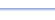Program: NTHTERM

NTHTERM

• Calculates the nth term of an arithmetic or geometric sequence.

Useful for:

• SAT
• ACT
• Algebra 2
• Precalculus
• Calculus, especially AP Calculus BC

See full list of programs

Try these problems using NTHTERM:
on MathPro Q&A

Example 1

Q: The first term of a geometric sequence is 5.  Each successive term is obtained by dividing the previous term by 3.  What is the sixth term?

Solution:  Run NTHTERM.  How?

Select choice 2
for 'Geometric'.

Enter the given
values as shown:

The program reports:

Interpretation:
The sixth term is 5/243.

See Example 2---------------->